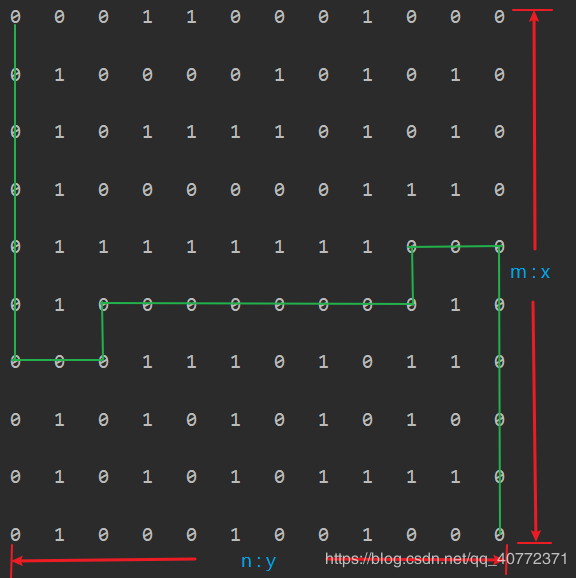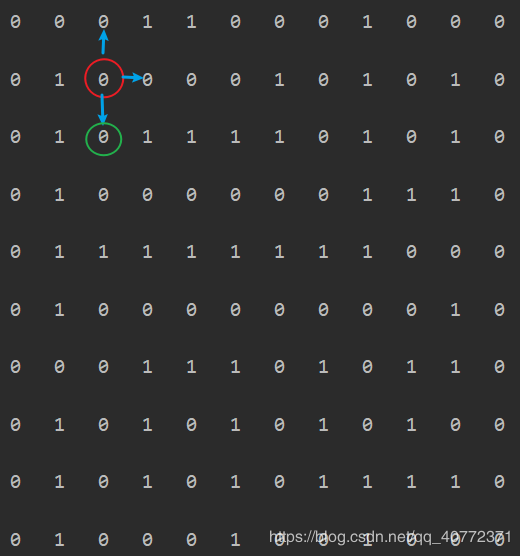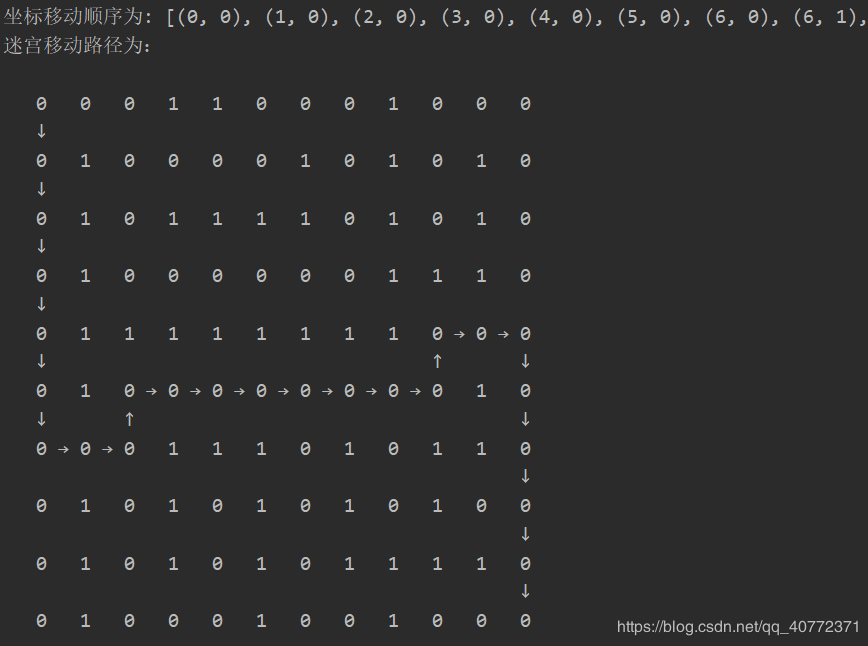欢迎访问优讯网！
 您当前的位置：首页 > 爱编程

# Python 实现递归法解决迷宫问题的示例代码• m：对应
• x 轴n：对应 y 轴
• 绿色线代表期望输出的路径

1. 标记当前所在位置
2. 如果此时所在位置为终点，说明可以到达终点，退出递归；1234567 `def` `mark(maze, pos):``  ``"""``  ``标记函数，用来标记历史走过的位置``  ``:param maze: 一个 m*n 大小的二维矩阵迷宫``  ``:param pos: 当前需要标记的位置坐标 pos = (x, y)，x = pos, y = pos``  ``"""``  ``maze[pos[``0``]][pos[``1``]] ``=` `2` `# 将走过的位置标记为 2`

 12345678 `def` `move(maze, pos):``  ``"""``  ``移动函数，用来测试当前位置是否可继续移动，移动条件为当前位置为 0``  ``:param maze: 一个 m*n 大小的二维矩阵迷宫``  ``:param pos: 当前需要标记的位置坐标 pos = (x, y)，x = pos, y = pos``  ``:return: bool 类型``  ``"""``  ``return` `maze[pos[``0``]][pos[``1``]] ``=``=` `0`

 123456789101112131415161718192021222324252627 `def` `find_path(maze, start, end):``  ``"""``  ``路径查找函数``  ``:param maze: 一个 m*n 大小的二维矩阵迷宫``  ``:param start: 起始点位置坐标，start = (1, 1)``  ``:param end: 终点坐标，end = (m, n)``  ``:return: bool 类型``  ``"""``  ``mark(maze, start) ``# 将起始位置标记``  ``if` `start ``=``=` `end: ``# 路径查找（递归）终止条件为到达终点``    ``move_path.append(start)``    ``return` `True` `  ``# 未到达终点时，存在 4 种可能的移动方向，即上 (-1, 0)，下 (1, 0)，左 (0, -1)，右 (0, 1)``  ``move_direction ``=` `[``    ``(``-``1``, ``0``), (``1``, ``0``), (``0``, ``-``1``), (``0``, ``1``)``  ``]``  ``direction ``=` `[``'↑'``, ``'↓'``, ``'←'``, ``'→'``]``  ``for` `i ``in` `range``(``4``): ``# 遍历 4 种可能的方向``    ``next_start ``=` `(start[``0``] ``+` `move_direction[i][``0``], start[``1``] ``+` `move_direction[i][``1``])``# 下一个可能的起始点坐标``    ``if` `move(maze, next_start): ``# 找出存在 0 即可移动的下一个起始点坐标，进入递归``      ``if` `find_path(maze, next_start, end):``        ``# 这里之所以仍然添加起始点坐标是因为当查询到下一个位置就是终点或者可到达终点时记录此时位置``        ``move_path.append(start)``        ``path_direction.append(direction[i]) ``# 记录路径方向``        ``return` `True``  ``return` `False` `# 遍历递归了 4 种可能方向后仍不能到达终点则说明无法走出迷宫`

 123456789101112131415161718192021222324252627282930313233 `def` `path_maze(maze, directions_map):``  ``"""``  ``生成带有移动路径的迷宫矩阵``  ``:param maze: 一个 m*n 大小的二维矩阵迷宫``  ``:param directions_map: 一个记录移动方向坐标的字典，有 ↑，↓，←，→ 4 个元素``  ``:return: path_maze``  ``"""``  ``n, m ``=` `len``(maze[``0``]), ``len``(maze)``  ``for` `x ``in` `range``(``1``, m``-``1``):``    ``for` `y ``in` `range``(``1``, n``-``1``):``      ``maze[x][y] ``=` `maze[x][y] ``if` `maze[x][y] !``=` `2` `else` `0` `# 将标记的 2 还原为 0` `  ``for` `x ``in` `range``(m):``    ``for` `i ``in` `range``(``1``, ``2` `*` `n ``-` `1``, ``2``):``      ``maze[x].insert(i, ``'  '``)``# 重初始化 maze，在每两个元素间插入占位符 '  ' 3 个空格` `  ``for` `x ``in` `range``(``1``, ``2` `*` `m ``-` `1``, ``2``):``    ``maze.insert(x, [``' '``, ``'  '``] ``*` `(n``-``1``) ``+` `['``']) # 插入两种空格占位符 '` `' 和 '`  `'` `  ``for` `direction ``in` `directions_map:``    ``for` `directions_position ``in` `directions_map[direction]:``      ``i, j ``=` `directions_position``      ``i ``=` `2` `*` `i``      ``j ``=` `2` `*` `j``      ``if` `direction ``=``=` `"↑"``:``        ``maze[i ``-` `1``][j] ``=` `"↑"``      ``if` `direction ``=``=` `"↓"``:``        ``maze[i ``+` `1``][j] ``=` `"↓"``      ``if` `direction ``=``=` `"←"``:``        ``maze[i][j] ``=` `" ← "``      ``if` `direction ``=``=` `"→"``:``        ``maze[i][j ``+` `1``] ``=` `" → "``  ``return` `maze`123456789101112131415161718 `def` `print_maze(maze, text``=``'原始迷宫为：'``, end1``=``'  '``, end2``=``'\n\n'``, xs``=``0``, xe``=``0``, ys``=``0``, ye``=``0``):``  ``"""``  ``输出迷宫矩阵，非必要，可注释删除``  ``:param maze: 一个 m*n 大小的二维矩阵迷宫``  ``:param text: 输出提示``  ``:param end1: 控制每行尾结束符``  ``:param end2: 控制每行尾结束符``  ``:param xs: 控制是否输出最上方的 1 环，0 为输出，1 为不输出``  ``:param xe: 控制是否输出最上方的 1 环，0 为输出，1 为不输出``  ``:param ys: 控制是否输出最上方的 1 环，0 为输出，1 为不输出``  ``:param ye: 控制是否输出最上方的 1 环，0 为输出，1 为不输出``  ``"""``  ``print``(text)``  ``n, m ``=` `len``(maze[``0``]), ``len``(maze)``  ``for` `x ``in` `range``(xs, m``-``xe):``    ``for` `y ``in` `range``(ys, n``-``ye):``      ``print``(maze[x][y], end``=``end1)``    ``print``(end``=``end2)`123456789101112131415161718192021222324252627282930313233343536373839404142434445464748495051525354555657585960616263646566676869707172737475767778798081828384858687888990919293949596979899100101102103104105106107108109110111112113114115116117118119120121122123124125126127128129130131132133134135136137138139140141142143144145146147148149150151152153154155156157158159160161162163164165166167168169170171172173174175176177178179180181182183184185186187188189 `# -*- coding: utf-8 -*-``"""``Created on 2020/1/11 10:51``Author : zxt``File  : maze_recursion.py``Software: PyCharm``"""`  `from` `random ``import` `randint`  `def` `mark(maze, pos):``  ``"""``  ``标记函数，用来标记历史走过的位置``  ``:param maze: 一个 m*n 大小的二维矩阵迷宫``  ``:param pos: 当前需要标记的位置坐标 pos = (x, y)，x = pos, y = pos``  ``"""``  ``maze[pos[``0``]][pos[``1``]] ``=` `2` `# 将走过的位置标记为 2`  `def` `move(maze, pos):``  ``"""``  ``移动函数，用来测试当前位置是否可继续移动，移动条件为当前位置为 0``  ``:param maze: 一个 m*n 大小的二维矩阵迷宫``  ``:param pos: 当前需要标记的位置坐标 pos = (x, y)，x = pos, y = pos``  ``:return: bool 类型``  ``"""``  ``return` `maze[pos[``0``]][pos[``1``]] ``=``=` `0`  `move_path ``=` `[] ``# 记录能成功到达出口的移动路径坐标``path_direction ``=` `[] ``# 记录能成功到达出口的移动路径方向`  `def` `find_path(maze, start, end):``  ``"""``  ``路径查找函数``  ``:param maze: 一个 m*n 大小的二维矩阵迷宫``  ``:param start: 起始点位置坐标，start = (1, 1)``  ``:param end: 终点坐标，end = (m, n)``  ``:return: bool 类型``  ``"""``  ``mark(maze, start) ``# 将起始位置标记``  ``if` `start ``=``=` `end: ``# 路径查找（递归）终止条件为到达终点``    ``move_path.append(start)``    ``return` `True` `  ``# 未到达终点时，存在 4 种可能的移动方向，即上 (-1, 0)，下 (1, 0)，左 (0, -1)，右 (0, 1)``  ``move_direction ``=` `[``    ``(``-``1``, ``0``), (``1``, ``0``), (``0``, ``-``1``), (``0``, ``1``)``  ``]``  ``direction ``=` `[``'↑'``, ``'↓'``, ``'←'``, ``'→'``]``  ``for` `i ``in` `range``(``4``): ``# 遍历 4 种可能的方向``    ``next_start ``=` `(start[``0``] ``+` `move_direction[i][``0``], start[``1``] ``+` `move_direction[i][``1``]) ``# 下一个可能的起始点坐标``    ``if` `move(maze, next_start): ``# 找出存在 0 即可移动的下一个起始点坐标，进入递归``      ``if` `find_path(maze, next_start, end):``        ``# 这里之所以仍然添加起始点坐标是因为当查询到下一个位置就是终点或者可到达终点时记录此时位置``        ``move_path.append(start)``        ``path_direction.append(direction[i]) ``# 记录路径方向``        ``return` `True``  ``return` `False` `# 遍历递归了 4 种可能方向后仍不能到达终点则说明无法走出迷宫`  `def` `gen_maze(m, n):``  ``"""``  ``生成随机迷宫阵列``  ``:param m: int 类型``  ``:param n: int 类型``  ``:return: maze``  ``"""``  ``m ``+``=` `2``  ``n ``+``=` `2` `# m 和 n 均 +2 是为了构造最外层的 1``  ``maze ``=` `[[``1` `for` `i ``in` `range``(n)] ``for` `j ``in` `range``(m)] ``# 初始化大小为 m * n，值全为 1 的二维矩阵``  ``for` `x ``in` `range``(``1``, m``-``1``):``    ``for` `y ``in` `range``(``1``, n``-``1``):``      ``"""``      ``这里 x, y 取值范围为 x ∈ [1, m-1)，y ∈ [1, n-1)是因为我们令此迷宫的最外层（四周）均为 1，如：``      ``考察 3 * 3 矩阵，一种可能的阵列为：``      ``[``       ``_ |←--- n:y ---→|``       ``↑ [1, 1, 1, 1, 1],``       ``| [1, 0, 1, 0, 1],``      ``m:x [1, 0, 0, 1, 1],``       ``| [1, 1, 0, 0, 1],``       ``↓ [1, 1, 1, 1, 1] ``      ``]``      ``"""``      ``if` `(x ``=``=` `1` `and` `y ``=``=` `1``) ``or` `(x ``=``=` `m ``-` `2` `and` `y ``=``=` `n ``-` `2``):``        ``maze[x][y] ``=` `0` `# 起始点和终点必为 0``      ``else``:``        ``maze[x][y] ``=` `randint(``0``, ``1``) ``# 在最外层均为 1 的情况下内部随机取 0，1``  ``return` `maze`  `def` `print_maze(maze, text``=``'原始迷宫为：'``, end1``=``'  '``, end2``=``'\n\n'``, xs``=``0``, xe``=``0``,ys``=``0``, ye``=``0``):``  ``"""``  ``输出迷宫矩阵，非必要，可注释删除``  ``:param maze: 一个 m*n 大小的二维矩阵迷宫``  ``:param text: 输出提示``  ``:param end1: 控制每行尾结束符``  ``:param end2: 控制每行尾结束符``  ``:param xs: 控制是否输出最上方的 1 环，0 为输出，1 为不输出``  ``:param xe: 控制是否输出最上方的 1 环，0 为输出，1 为不输出``  ``:param ys: 控制是否输出最上方的 1 环，0 为输出，1 为不输出``  ``:param ye: 控制是否输出最上方的 1 环，0 为输出，1 为不输出``  ``"""``  ``print``(text)``  ``n, m ``=` `len``(maze[``0``]), ``len``(maze)``  ``for` `x ``in` `range``(xs, m``-``xe):``    ``for` `y ``in` `range``(ys, n``-``ye):``      ``print``(maze[x][y], end``=``end1)``    ``print``(end``=``end2)`  `def` `path_maze(maze, directions_map):``  ``"""``  ``生成带有移动路径的迷宫矩阵``  ``:param maze: 一个 m*n 大小的二维矩阵迷宫``  ``:param directions_map: 一个记录移动方向坐标的字典，有 ↑，↓，←，→ 4 个元素``  ``:return: path_maze``  ``"""``  ``n, m ``=` `len``(maze[``0``]), ``len``(maze)``  ``for` `x ``in` `range``(``1``, m``-``1``):``    ``for` `y ``in` `range``(``1``, n``-``1``):``      ``maze[x][y] ``=` `maze[x][y] ``if` `maze[x][y] !``=` `2` `else` `0` `# 将标记的 2 还原为 0` `  ``for` `x ``in` `range``(m):``    ``for` `i ``in` `range``(``1``, ``2` `*` `n ``-` `1``, ``2``):``      ``maze[x].insert(i, ``'  '``) ``# 重初始化 maze，在每两个元素间插入占位符'  ' 3 个空格` `  ``for` `x ``in` `range``(``1``, ``2` `*` `m ``-` `1``, ``2``):``    ``maze.insert(x, [``' '``, ``'  '``] ``*` `(n``-``1``) ``+` `['``']) # 插入两种空格占位符 '` `' 和 '`  `'` `  ``for` `direction ``in` `directions_map:``    ``for` `directions_position ``in` `directions_map[direction]:``      ``i, j ``=` `directions_position``      ``i ``=` `2` `*` `i``      ``j ``=` `2` `*` `j``      ``if` `direction ``=``=` `"↑"``:``        ``maze[i ``-` `1``][j] ``=` `"↑"``      ``if` `direction ``=``=` `"↓"``:``        ``maze[i ``+` `1``][j] ``=` `"↓"``      ``if` `direction ``=``=` `"←"``:``        ``maze[i][j] ``=` `" ← "``      ``if` `direction ``=``=` `"→"``:``        ``maze[i][j ``+` `1``] ``=` `" → "``  ``return` `maze`  `def` `main():``  ``# maze = gen_maze(m=10, n=12)``  ``maze ``=` `\``    ``[``      ``[``1``, ``1``, ``1``, ``1``, ``1``, ``1``, ``1``, ``1``, ``1``, ``1``, ``1``, ``1``, ``1``, ``1``],``      ``[``1``, ``0``, ``0``, ``0``, ``1``, ``1``, ``0``, ``0``, ``0``, ``1``, ``0``, ``0``, ``0``, ``1``],``      ``[``1``, ``0``, ``1``, ``0``, ``0``, ``0``, ``0``, ``1``, ``0``, ``1``, ``0``, ``1``, ``0``, ``1``],``      ``[``1``, ``0``, ``1``, ``0``, ``1``, ``1``, ``1``, ``1``, ``0``, ``1``, ``0``, ``1``, ``0``, ``1``],``      ``[``1``, ``0``, ``1``, ``0``, ``0``, ``0``, ``0``, ``0``, ``0``, ``1``, ``1``, ``1``, ``0``, ``1``],``      ``[``1``, ``0``, ``1``, ``1``, ``1``, ``1``, ``1``, ``1``, ``1``, ``1``, ``0``, ``0``, ``0``, ``1``],``      ``[``1``, ``0``, ``1``, ``0``, ``0``, ``0``, ``0``, ``0``, ``0``, ``0``, ``0``, ``1``, ``0``, ``1``],``      ``[``1``, ``0``, ``0``, ``0``, ``1``, ``1``, ``1``, ``0``, ``1``, ``0``, ``1``, ``1``, ``0``, ``1``],``      ``[``1``, ``0``, ``1``, ``0``, ``1``, ``0``, ``1``, ``0``, ``1``, ``0``, ``1``, ``0``, ``0``, ``1``],``      ``[``1``, ``0``, ``1``, ``0``, ``1``, ``0``, ``1``, ``0``, ``1``, ``1``, ``1``, ``1``, ``0``, ``1``],``      ``[``1``, ``0``, ``1``, ``0``, ``0``, ``0``, ``1``, ``0``, ``0``, ``1``, ``0``, ``0``, ``0``, ``1``],``      ``[``1``, ``1``, ``1``, ``1``, ``1``, ``1``, ``1``, ``1``, ``1``, ``1``, ``1``, ``1``, ``1``, ``1``]``    ``] ``# 输入样式矩阵，这里最外层用 1 环包围住，目的是方便后续的处理，可以用 gen_maze() 函数自生成``  ``print_maze(maze)``  ``if` `find_path(maze, start``=``(``1``, ``1``), end``=``(``10``, ``12``)):``    ``mp ``=` `move_path[::``-``1``]``    ``pd ``=` `path_direction[::``-``1``]``    ``# 这里 pos 和 pos 都要 -1 是因为原来的递归计算中存在最外层的 1 环``    ``print``(``'坐标移动顺序为:'``, [(pos[``0``]``-``1``, pos[``1``]``-``1``) ``for` `pos ``in` `mp])``    ``path_direction_map ``=` `{``      ``'↑'``: [],``      ``'↓'``: [],``      ``'←'``: [],``      ``'→'``: []``    ``} ``# 路径方向的映射表``    ``for` `i ``in` `range``(``len``(pd)):``      ``path_direction_map[pd[i]].append(mp[i])``    ``maze ``=` `path_maze(maze, path_direction_map)``    ``print_maze(maze, text``=``'迷宫移动路径为：'``, end1``=``'``', end2='``\n',xs``=``1``, xe``=``1``, ys``=``1``, ye``=``1``)``  ``else``:``    ``print``(``'此迷宫无解'``)`  `if` `__name__ ``=``=` `'__main__'``:``  ``main()`

以上就是本文的全部内容，希望对大家的学习有所帮助返回首页
 原文链接：https://www.jb51.net/article/178284.htm
 推荐资讯
 相关文章
 栏目更新
 栏目热门